# NCERT Solutions for Class 10 Maths Areas Related to Circles Exercise 12.3

In this page we have NCERT Solutions for Class 10 Maths Areas Related to Circles for Exercise 12.3 . Hope you like them and do not forget to like , social share and comment at the end of the page.
Unless stated otherwise, use π =22/7
Question 1
Find the area of the shaded region in below figure, if PQ = 24 cm, PR = 7 cm and O is the centre of the circle.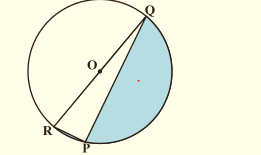Solution
PQ = 24 cm and PR = 7 cm
∠P = 90° (Angle in the semi-circle)
By Pythagoras theorem,
QR= PR2  + PQ2
QR= 72  + 242
QR = 25 cm
Now Diameter of the circle=QR
Therefore, Radius of the circle = 25/2 cm
Area of the semicircle = (π R2)/2
= (22/7 × 25/2 × 25/2)/2 cm2
= 13750/56 cm= 245.54 cm2
Area of the ΔPQR = 1/2 × PR × PQ
= 1/2 × 7 × 24 cm2
= 84 cm2
Area of the shaded region = 245.54 - 84   = 161.54 cm2

Question 2
Find the area of the shaded region in below figure, if radii of the two concentric circles with centre O are 7 cm and 14 cm respectively and ∠AOC = 40°.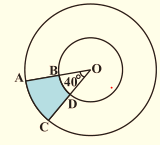Solution
Radius inner circle = 7 cm
Radius of outer circle = 14 cm
Angle made by sector = 40°
Area of the sector OAC = (40°/360°) × π r
= 1/9 × 22/7 × 14= 68.44 cm2
Area of the sector OBD = (40°/360°) × π r
= 1/9 × 22/7 × 72  = 17.11 cm2

Area of the shaded region ABDC = Area of the sector OAC - Area of the sector circle OBD
= 68.44  - 17.11 = 51.33 cm2

Question 3
Find the area of the shaded region in below figure, if ABCD is a square of side 14 cm and APD and BPC are semicircles.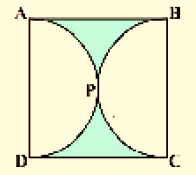Solution
There are two semicircles in the figure.
Side of the square = 14 cm
Therefore, Diameter of the semicircle = 14 cm
Radius of the semicircle = 7 cm
Area of the square = 14 × 14 = 196 cm2
Area of the semicircle = (π R2)/2
= (22/7 × 7 × 7)/2 cm= 77 cm
Area of two semicircles = 2 × 77 = 154 cm2

Area of the shaded region = 196 154 = 42 cm2

Question 4
Find the area of the shaded region in below figure, where a circular arc of radius 6 cm has been drawn with vertex O of an equilateral triangle OAB of side 12 cm as centre.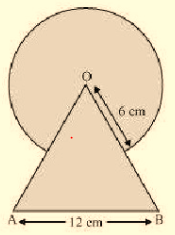Solution
OAB is an equilateral triangle with each angle equal to 60°.
Area of the sector is common in both.
Radius of the circle = 6 cm.
Side of the triangle = 12 cm.
Area of the equilateral triangle = √3/4 × (OA)= √3/4 × 12= 36√3 cm2
Area of the circle = π R2 = 22/7 × 6= 792/7 cm2
Area of the sector making angle 60° = (60°/360°) × π r
= 1/6 × 22/7 × 62= 132/7 cm2
Area of the shaded region = Area of the equilateral triangle + Area of the circle - Area of the sector
= 36√3 + 792/7 - 132/7
= (36√3 + 660/7) cm2

Question 5
From each corner of a square of side 4 cm a quadrant of a circle of radius 1 cm is cut and a circle of diameter 2 cm is cut as shown in below figure. Find the area of the remaining portion of the square.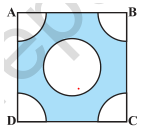Solutions
Side of the square = 4 cm
Radius of the circle = 1 cm
Four quadrant of a circle are cut from corner and one circle of radius are cut from middle.
Area of square = (side)= 4= 16 cm2
Area of the quadrant = (π R2)/4 cm2 = (22/7 × 12)/4 = 11/14 cm2
Therefore, Total area of the 4 quadrants = 4 × (11/14) cm2 = 22/7 cm2
Area of the circle = π Rcm2 = (22/7 × 12) = 22/7 cm2
Area of the shaded region = Area of square - (Area of the 4 quadrants + Area of the circle)
= 16 cm- (22/7 + 22/7) cm2
= 68/7 cm2

Question 6
In a circular table cover of radius 32 cm, a design is formed leaving an equilateral triangle ABC in the middle as shown in below figure. Find the area of the design.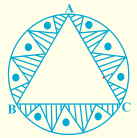Solution
Radius of the circle = 32 cm
Draw a median AD of the triangle passing through the centre of the circle.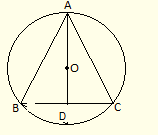Since, AD is the median of the triangle
By Pythagoras theorem,
AB= 482   + (AB/2)2   ( As BD is the mid-point )
3/4 (AB2) = 2304
AB = 32√3 cm
Area of ΔABC = √3/4 × (32√3)= 768√3 cm2
Area of circle = π R2 = 22/7 × 32 × 32 = 22528/7 cm2

Area of the design = Area of circle - Area of ΔBC
= (22528/7 - 768√3) cm2
Question 7
In below figure, ABCD is a square of side 14 cm. With centers A, B, C and D, four circles are drawn such that each circle touch externally two of the remaining three circles. Find the area of the shaded region.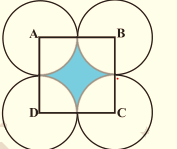Solution
Side of square = 14 cm
Four quadrants are included in the four sides of the square.
Therefore, Radius of the circles = 14/2 cm = 7 cm
Area of the square ABCD = 14= 196 cm2
Area of the quadrant = (π R2)/4 = (22/7 × 72)/4 cm2
= 77/2 cm2
Total area of the quadrant = 4 × 77/2 cm= 154 cm

Area of the shaded region = Area of the square ABCD - Area of the quadrant
= 196 - 154
= 42 cm
Question 8
Below Figure depicts a racing track whose left and right ends are semicircular. The distance between the two inner parallel line segments is 60 m and they are each 106 m long. If the track is 10 m wide, find
(i) the distance around the track along its inner edge
(ii) the area of the track.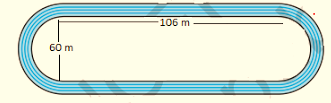Solution
Width of track = 10 m
Distance between two parallel lines = 60 m
Length of parallel tracks = 106 m
DE = CF = 60 m
Radius of inner semicircle, r = OD = O'C
= 60/2 m = 30 m
Radius of outer semicircle, R = OA = O'B
= 30 + 10 m = 40 m
Also, AB = CD = EF = GH = 106 m
Distance around the track along its inner edge = CD + EF + 2 × (Circumference of inner semicircle)
= 106 + 106 + (2 × πr) m = 212 + (2 × 22/7 × 30) m
= 2804/7 m

Area of the track = Area of ABCD + Area EFGH + 2 × (area of outer semicircle) - 2 × (area of inner semicircle)
= (AB × CD) + (EF × GH) + 2 × (πr2/2) - 2 × (πR2/2) m2
= (106 × 10) + (106 × 10) + 2 × π/2 (r2 -R2) m2
= 4320 m

Question 9
In below figure, AB and CD are two diameters of a circle (with centre O) perpendicular to each other and OD is the diameter of the smaller circle. If OA = 7 cm, find the area of the shaded region.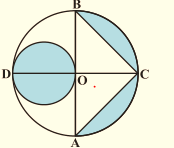Solution
Radius of larger circle, R = 7 cm
Radius of smaller circle, r = 7/2 cm
Height of ΔBCA = OC = 7 cm
Base of ΔBCA = AB = 14 cm
Area of ΔBCA = 1/2 × AB × OC = 1/2 × 7 × 14 = 49 cm
Area of larger circle = πR= 22/7 × 72 = 154 cm
Area of larger semicircle = 154/2 cm= 77 cm
Area of smaller circle = πr2 = 22/7 × 7/2 × 7/2 = 38.5 cm2

= Area of larger circle - Area of triangle - Area of larger semicircle + Area of smaller circle
Area of the shaded region = (154 - 49 - 77 + 39.5)
= 66.5 cm2

Question 10
The area of an equilateral triangle ABC is 17320.5 cm2. With each vertex of the triangle as centre, a circle is drawn with radius equal to half the length of the side of the triangle. Find the area of the shaded region. (Use π = 3.14 and √3 = 1.73205)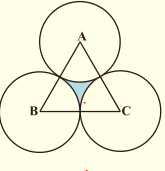Solution
ABC is an equilateral triangle.
Therefore, ∠A = ∠B = ∠C = 60°
There are three sectors each making 60°.
Let x be the side of the equilateral triangle
Area of ΔABC = 17320.5 cm2
√3/4 × (x)= 17320.5
x = 200 cm
Therefore, Radius of the circles = 200/2 cm = 100 cm
Area of the sector = (60°/360°) × π rcm2
= 1/6 × 3.14 × (100)cm2
= 15700/3 cm2

Area of the shaded region = Area of equilateral triangle ABC – 3 × Area of sector
= 17320.5 - 15700 cm= 1620.5 cm2

Question 11
On a square handkerchief, nine circular designs each of radius 7 cm are made . Find the area of the remaining portion of the handkerchief.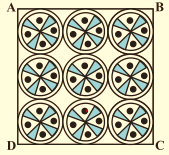Solution
Number of circular design = 9
Radius of the circular design = 7 cm
There are three circles in one side of square handkerchief.
Therefore, Side of the square = 3 × diameter of circle = 3 × 14 = 42 cm
Area of the square = 42 × 42 cm2 = 1764 cm2

Area of the circle = π r= 22/7 × 7 × 7 = 154 cm2
Area of the remaining portion of the handkerchief
= Area of the square - Total area of the design
= Area of the square - 9× Area of the circle
= 1764 - 1386 = 378 cm2

Question 12
In below figure, OACB is a quadrant of a circle with centre O and radius 3.5 cm. If OD = 2 cm, find the area of the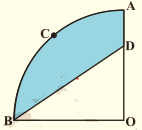Solution
(i) Area of quadrant OACB = (πR2)/4 cm2
= (22/7 × 3.52)/4 cm2
= 9.625 cm2
(ii) Area of triangle BOD = 1/2 × 3.5 × 2 cm2
= 3.5 cm2
Area of shaded region = Area of quadrant - Area of triangle BOD
= (9.625 – 3.5) cm2
= 6.125 cm2
Question 13
In below figure, a square OABC is inscribed in a quadrant OPBQ. If OA = 20 cm, find the area of the shaded region. (Use π = 3.14)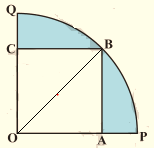Solution
Side of square = OA = AB = 20 cm
Now OB is the diagonal of the square
OB = 20√2 cm
Area of the quadrant = (πR2)/4 = 3.14/4 × (20√2)= 628 cm2
Area of the square = 20 × 20 = 400   cm2

Area of the shaded region = Area of the quadrant - Area of the square
= 628 - 400 = 228 cm2

Question 14
AB and CD are respectively arcs of two concentric circles of radii 21 cm and 7 cm and centre O .If ∠AOB = 30°, find the area of the shaded region.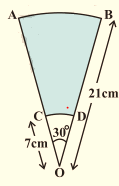Solution
Radius of the larger circle, R = 21 cm
Radius of the smaller circle, r = 7 cm
Angle made by sectors of both concentric circles = 30°
Area of the larger sector = (30°/360°) × π Rcm2
= 1/12 × 22/7 × 21cm2
= 231/2 cm2
Area of the smaller circle = (30°/360°) × π rcm2
= 1/12 × 22/7 × 7cm2
= 77/6 cm2

Area of the shaded region = 231/2 - 77/6 cm2
= 616/6 cm2 = 308/3 cm2

Question 15
In below figure, ABC is a quadrant of a circle of radius 14 cm and a semicircle is drawn with BC as diameter. Find the area of the shaded region.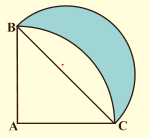Solution
AB = AC = 14 cm
BC is diameter of semicircle.
ABC is right angled triangle.
By Pythagoras theorem in ΔABC,
BC= AB2  + AC2
BC= 142  + 142
BC = 14√2 cm
Radius of semicircle = 14√2/2 cm = 7√2 cm
Area of ΔABC = 1/2 × 14 × 14 = 98 cm2
Area of quadrant = 1/4 × 22/7 × 14 × 14 = 154 cm2
Area of the semicircle = 1/2 × 22/7 × 7√2 × 7√2 = 154 cm2

Area of the shaded region = Area of the semicircle –(Area of quadrant - Area of ΔABC )
= 154 + 98 - 154 cm= 98 cm2

Question 16
Calculate the area of the designed region in below figure common between the two quadrants of circles of radius 8 cm each.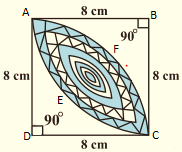Solution
AB = BC = CD = AD = 8 cm
Area of ΔABC = Area of ΔADC = 1/2 × 8 × 8 = 32 cm2
Area of quadrant AECB = Area of quadrant AFCD = 1/4 × 22/7 × 82
= 352/7 cm2

(Area of quadrant AECB - Area of ΔABC) +  (Area of quadrant AFCD - Area of ΔADC)
= (352/7 - 32) + (352/7 -32) cm2
= 2 × (352/7 -32) cm2
=  256/7 cm2Go back to Class 10 Main Page using below links

### Practice Question

Question 1 What is $1 - \sqrt {3}$ ?
A) Non terminating repeating
B) Non terminating non repeating
C) Terminating
D) None of the above
Question 2 The volume of the largest right circular cone that can be cut out from a cube of edge 4.2 cm is?
A) 19.4 cm3
B) 12 cm3
C) 78.6 cm3
D) 58.2 cm3
Question 3 The sum of the first three terms of an AP is 33. If the product of the first and the third term exceeds the second term by 29, the AP is ?
A) 2 ,21,11
B) 1,10,19
C) -1 ,8,17
D) 2 ,11,20